# LabVIEW

cancel
Showing results for
Did you mean:

## Dividing in FPGA?

Does anyone know a method of dividing in labview FPGA without using the remainder and quotient function?

The normal divide function is not supported in labview FPGA and the quotient and remainder function introduces a constant delay.
I've tried ouputing the parameters and performing the division in the real time environment (where normal divide is supported) however this introduces a delay at high frequency due to jitter.
Message 1 of 9
(15,518 Views)

## Re: Dividing in FPGA?

You can do the same thing as the remainder and quotient function by subtracting the two numbers in a while loop.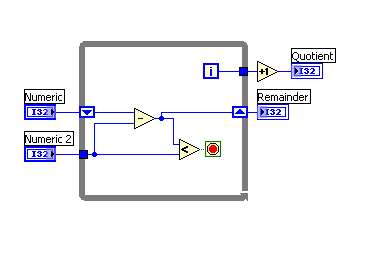I'm not sure if this will be any better than the remainder and quotient function, but I can't think of any other way.

Ed

Message Edited by Ed Dickens on 03-04-2005 08:21 AM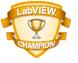Ed Dickens - Certified LabVIEW Architect - DISTek Integration, Inc. - NI Certified Alliance Partner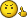Using the Abort button to stop your VI is like using a tree to stop your car. It works, but there may be consequences.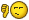Message 2 of 9
(15,510 Views)

## Re: Dividing in FPGA?

An efficient method to handle division or any kind of non-integer scaling is to use a Multiply and Scale by Power of 2 function. In addition by wrapping them in a Single Cycle Timed Loop in LabVIEW FPGA you will get optimal performance out of these functions.

The Scaling Constant and Shift value will determine the value by which you are multiplying your numerator. For example to divide by 2, set the Scaling constant to 1 and the Shift to -1. To divide by four set the Scaling constant to 1 and the Shift to -2.

You have to make sure that the result of the multiplication can still fit within the data type you are using. With the Saturation Multiply function you can multiply two 16-bit integers and be sure that the result will fit into a 32-bit integer. Of course if your result needs to be a 16- bit integer then the Scale by Power of 2 function needs to shift the product back into the 16-bit range. This method will not give you exact results for every scaling value, but it will give you very good approximations. The trick is to find the right combination of Scaling Constant and Shift value.

To divide by 10, you can use a Scaling constant of 102 and a shift of -10. In effect you are multiplying your numerator by 102 and then dividing by 1024. 102/1024 = 0.099609375 = 1/10.039

To find the right Scaling Constant and Shift value, it is often easiest to pick a suitably large Shift value and then calculate the corresponding Scaling Constant for your needs.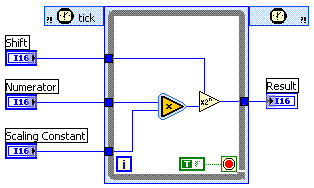Message Edited by Christian L on 03-04-2005 04:55 PM

authored by
Christian L, CLA
Systems Engineering Manager - Automotive and Transportation
NI - Austin, TX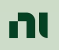Message 3 of 9
(15,497 Views)

## Re: Dividing in FPGA?

The attached VI shows a simple example on how to calculate the Scaling Constant and Shift value for my previous post. This code will be run as part of the host application in Windows or LabVIEW Realtime and assumes you will be using an I16 Numerator. It uses an I32 intermediate value to get the best result.

Message Edited by Christian L on 03-04-2005 05:10 PM

authored by
Christian L, CLA
Systems Engineering Manager - Automotive and Transportation
NI - Austin, TXMessage 4 of 9
(15,479 Views)

## Re: Dividing in FPGA?

I see that the host VI is calculating the scaling factors automatically without the need of the user to enter the bit shift and multiplier. Can this be implemented onto the FPGA VI target?
Message 5 of 9
(14,340 Views)

## Re: Dividing in FPGA?

can you tell the reason behind, "divide is not working in FPGA"?

Message 6 of 9
(8,374 Views)

## Re: Dividing in FPGA?

Hi Shiva,

you are asking in a 13 years old thread: quite a lot has changed in this time…

Surely the Divide operation is supported in the FPGA, even with SGL data since several years. BUT: a division (most often) requires a lot of resources in the FPGA!

So the recommendation is: avoid division, when possible…

Message 7 of 9
(8,365 Views)

## Re: Dividing in FPGA?

"a division (most often) requires a lot of resources in the FPGA!", still i couldn't get this gerd..

by the way, i'm new to the FPGA concepts..so, can you elaborate this thing (dividing in FGPA)?

Message 8 of 9
(8,355 Views)

## Re: Dividing in FPGA?

Hi Shiva,

in FPGA you can use most functions you also use in "standard" LabVIEW on "standard" computer.

THe difference is: in standard PC "heavy" computations need more time, but in the FPGA "heavy" operations consume more "fabric"/"space"/"resources".

Keep in mind: the FPGA is a piece of hardware providing a limited amount of "computational units" (aka resources), but those resources can execute all at the same speed (more or less)…

Message 9 of 9
(8,350 Views)